# Maximal term of a series

(diff) ← Older revision | Latest revision (diff) | Newer revision → (diff)

The term of a convergent series of numbers or functions with positive terms the value of which is not less than the values of all other terms of this series.

Applying this idea to the study of power series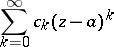in one complex variablewith positive radius of convergence,, one has in mind the maximal termof the seriesThus,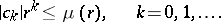The index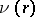of the maximal termis called the central index: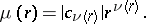If there are several terms in modulus equal to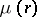, then the central index is taken to be the largest of the indices of these terms. The function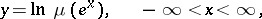is non-decreasing and convex; the functionis a step-function, increases at discontinuity points in natural numbers and is everywhere continuous from the right.

How to Cite This Entry:
Maximal term of a series. Encyclopedia of Mathematics. URL: http://encyclopediaofmath.org/index.php?title=Maximal_term_of_a_series&oldid=18824
This article was adapted from an original article by E.D. Solomentsev (originator), which appeared in Encyclopedia of Mathematics - ISBN 1402006098. See original article